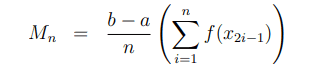# Midpoint Riemann sum approximation

• Leo Liu

#### Leo LiuCan someone please explain why the formula for midpoint approximation looks like the equation above instead of something like
$$M_n=(f(\frac{x_0+x_1}2)+f(\frac{x_1+x_2}2)+\cdots+f(\frac{x_{n-1}+x_n}2))\frac{b-a}n$$?
•